# McRuffy Math Blog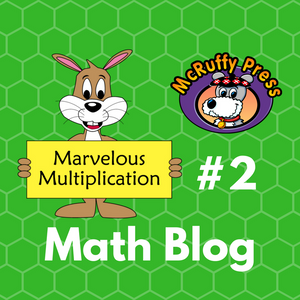## Marvelous Multiplication #2

Marvelous Multiplication Trick #2: A Multiplication Challenge Using Easy Squares.

If you haven’t already, check out our previous three blogs:

Quick Math: Find the Squares of Numbers That End With 5

Quick Math: Squaring Numbers 51 to 59

In the previous post (Marvelous Multiplication #1) we generated and easily solved problems based on what we termed “easy squares” of two-digit numbers. This included the techniques learned in the two Quick Math blogs and squares of multiples of ten (10, 20, 30, 40…). We generated multipliers by adding and subtracting 1 to and from the easy squares.

In this post, we’ll expand the number of multiplication problems that can be generated and suggest a math challenge game.

The Trick:

Generate 2 two-digit multipliers around an easy square by adding and subtracting the same number to and from the easy square. In the last post, we subtracted 1, but the trick works with adding and subtracting any number. Let’s use the easy square of 30 for an example and add and subtract 2 for the multipliers. We’ll call the number 2 the “distance” between either multiplier and the easy square.

30 -2 = 28 and 30 + 2 = 32 Our multipliers will be 28 and 32.

To quickly find the product of 28 x 32 find the product of the easy square (302 = 900) and subtract the square of the distance. (22 = 4)

28 x 32 = 900 – 4 = 896

Let’s try it with a “distance” of 3:

30 – 3 = 27 and 30 + 3 = 33 Our multipliers will be 27 and 33.

27 x 33 = 900 – 9 = 891

Click here for the Teaching Sheets PDF

Teaching Sheet 1 provides a form children can use to practice stepping through the process. Chose an easy square and write the digits in the boxes. Choose a “distance” number. Add and subtract the “distance” to generate multipliers for the problem.

Write the product of the easy square on the bottom row and the distance square. Subtract the distance squared to find the answer.

Challenge Game:

Given a two-digit number, generate a problem around an Easy Square and use the math tricks to solve the problem. The ultimate goal would be doing all the steps using mental math, but teaching sheets 2 and 3 present the steps involved in solving the problems to practice thinking through the steps.

For example, try the number 43.

You can make an easy square based problem around the Easy Square of 45. The distance between 43 and 45 is 2. (45 – 43 = 2) The second multiplier will be 47 (45 + 2 = 47). The problem generated will be 43 x 47.

The Easy Square of 45 is 2025. The Distance of 2 squared is 4.

2025 – 4 = 2021 so 43 x 47 = 2021

You could also make an easy square based problem around the Easy Square of 40. The distance between 43 and 40 is 3. (43 – 40 = 3) The second multiplier will be 37 (40 - 3 = 37). The problem generated will be 43 x 37.

Easy Square is 402 = 1600 and the Distance squared is 32 = 9

43 x 37 = 1600 – 9 = 1591

You could also make an easy square based problem around the Easy Square of 53. The distance between 43 and 53 is 10. The second multiplier will be 63. The problem generated will be 43 x 63.

The Easy Square is 532 = 2809 and the Distance squared is 102 = 100

43 x 63 = 2809 – 100 = 2709

Sheet 2 shows all the steps. Start with a random two-digit number and write it on the top line of the “Generate a Problem” section. Choose a nearby easy square. Write the number that is to be added or subtracted to equal the easy square. Next, write the easy square. Generate the second multiplier by doing the opposite operation to the Easy Square. Write the second multiplier in the boxes.

On the bottom row write the products of the squares of the Easy Square and Distance. Subtract for the answer to the generated multiplication problem.

Sheet 3 visually simplifies the process. The first section is used to write the multiplication problem starting with any given two-digit number. The ES boxes are for the Easy Square. The D boxes are for the Distance. Most of the time it will be a single digit distance, but it can be any number of digits. The sheet is set up for a distance with a maximum of two-digits. The far right boxes are for the squares of the Easy Square and Distance.

Encourage children to skip any boxes they can do mentally. For example, if they can write the product of the Easy Square directly in the far right boxes, skip the center box. If the distance and distance square are smaller numbers and easy to remember those boxes can be skipped.

Why it Works:

Let’s build an algebra problem. We’ll represent the Easy Square with the letter a and the “distance” with the letter b. We generated the multipliers by adding and subtracting the “distance” to and from an Easy Square.

(a – b)(a + b) = a2 + ab – ab – b2 =  a2 – b2

This is true for any “distance” even when it makes numbers negative and multipliers have more than two digits. For example, with the number 30 we can make the “distance” 200.

The multipliers are 230 and -170

Easy Square is 302 = 900 and The distance squared is 2002 = 40,000

230 x (-170) = 900 - 40,000 = -39,100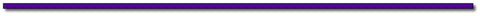## Thanks for Visting Savings:

Use the code MarMult2 to save \$3.00 off every Color Math Curriculum Package. See eligible products here.

Offer Expires July 30, 2017

Join our e-mail list!Subscribe to this blog's RSS feed using http://feeds.feedburner.com/McruffyPress-McruffyMathBlog## McRuffy Press offers complete math curriculum by grade level, math activity books, manipulatives, games and more! Find more McRuffy Math products here.

Improve multiplication facts skills with our Multiplication Fast Fact books: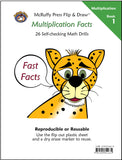Multiplication Facts (Book 1) Drills factors from 1 to 10 in our self-checking, non-consumable format that allows students to practice until they have mastered basic facts.Multiplication Practice (Book 2) Drills factors from 1 to 10 in our self-checking, non-consumable format that allows students to practice until they have mastered basic facts.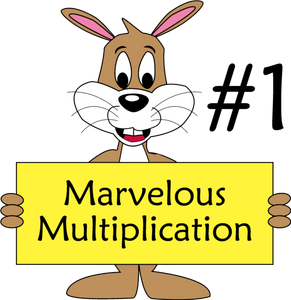## Marvelous Multiplication #1

Marvelous Multiplication Trick #1: Using Math Squaring Tricks to Multiply Other Numbers.

If you haven’t already, check out our previous two blogs:

Quick Math: Find the Squares of Numbers That End With 5

Quick Math: Squaring Numbers 51 to 59

We can use these techniques to do some Quick Math multiplying two-digit numbers that aren't squares. The tricks in this post offer more of an entertainment value than everyday practical value. Helping children feel empowered to do math is a great thing. These tricks do that! Once after teaching these techniques to some children, they replied, “Wow! That’s what algebra does.”

Like the previous two tricks, the minimum a child has to understand is basic multiplication facts. The Why it Works section is great to help students who have some understanding of algebra see how math mysteries can be resolved.

The Trick:

We’ll start with the example of 64 x 66. After learning the trick you’ll immediately see that the product is 4224. First, let’s explore lists of problems we can use for the trick.

We can apply the trick to all these problems:

14 x 16, 24 x 26, 34 x 36, 44 x 46, 54 x 56, 64 x 66, 74 x 76, 84 x 86, 94 x 96

Do you see the pattern?

It also applies to these problems:

50 x 52, 51 x 53, 52 x 54, 53 x 55, 54 x 56, 55 x 57, 56 x 58, 57 x 59, 58 x 60, 59 x 61

And these problems:

19 x 21, 29 x 31, 39 x 41, 49 x 51, 59 x 61, 69 x 71, 79 x 81, 89 x 91

The lists of problems do not need to be memorized because there is a simple method to generate the problems:

Start with a number that the child has learned to easily square. We’ll call a number to be squared an Easy Square.

The problems were generated by adding and subtracting one from the numbers that were squared. For example, start with the Easy Square of 65.

Generate the problem by subtracting and adding one: (65 – 1)(65 + 1) = 64 x 66.

The solution is simply the product of the Easy Square minus 1. Applying the Quick Math trick from the first blog we can quickly find the solution. 4225 – 1 = 4224.

The middle row of problems was generated from the Easy Squares demonstrated in the second blog.

For example, using the trick we can quickly know that 532 = 2809. From that we generate the problem 52 x 54 = 2809 – 1 = 2808

We can call the squares of two-digit numbers ending with zero Easy Squares, too. That was how we generated the last row of problems with 9’s and 1’s in the units place.

For example: 802 = 6400, so 79 x 81 = 6400 – 1 = 6399.

Practice the trick, and we’ll explore how to greatly expand the list of problems that can be generated in our next blog.

The Teaching Sheet provides a form children can use to practice stepping through the process. Chose an easy square and write the digits in the boxes. Skip to the other side of the page and generate a -1, +1 problem. Use an easy square trick to find the square of the Easy Square. Write the answer in the Th, H, T, O boxes. Subtract 1 to find the product of the generated problem.

Why it Works:

Let’s build an algebra problem. We’ll represent the Easy Square with the letter a. We generated the multipliers by adding and subtracting one to an Easy Square.

(a – 1)(a + 1) = a2 + a – a – 12 =  a2 – 1

The algebra problem shows we can substitute any of our Easy Square numbers. Apply the easy square trick and subtract one.

The formula is not limited to two-digit numbers. For example:

2002 = 40,000    So 199 x 201 = 39,999

Nor is it limited to Easy Squares. It’s just more difficult to apply. For example generating a problem from 392:

38 x 40 = 392 -1  = 1521 – 1 = 1520. It’s actually easier to multiply 38 x 40 than to first square 39 and then subtract one, so the trick just isn’t helpful. Nevertheless, there is a trick involving this problem, but that’s for another blog.## Thanks for Visting Free Gift:

Practice Multiplication Skills with Multiplication Sliders  Download Here and use the discount code: Marvelous#1 at checkout and receive your PDF file instantly!

Offer Expires July 15, 2017

Join our e-mail list!Subscribe to this blog's RSS feed using http://feeds.feedburner.com/McruffyPress-McruffyMathBlog## McRuffy Press offers complete math curriculum by grade level, math activity books, manipulatives, games and more! Find more McRuffy Math products here.

Improve multiplication facts skills with our Multiplication Fast Fact books:Multiplication Facts (Book 1) Drills factors from 1 to 10 in our self-checking, non-consumable format that allows students to practice until they have mastered basic facts.Multiplication Practice (Book 2) Drills factors from 1 to 10 in our self-checking, non-consumable format that allows students to practice until they have mastered basic facts.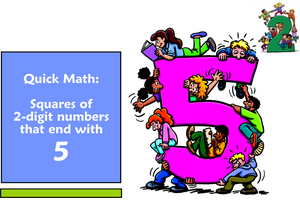## Quick Math: Find the Squares of Numbers That End With 5

Want to teach your child some amazing math shortcuts? Follow our new blog series. The first blog features a math trick to quickly square numbers that end with 5.

It’s simple enough for children who have knowledge of basic multiplication facts.

For younger children simply teach the trick. For students learning algebra, explore in the Why it Works section for the more mathematically curious. In future blogs, we’ll do some interesting things with this trick to multiply numbers that aren’t squares.

The Trick:

You can quickly find the square of any two digit number ending with 5, such as 15, 25, 35, 45, etc. The trick actually works for any number that ends with five, but the mental math becomes increasingly difficult with numbers greater than 95.

Simply look at the digit in the tens place and multiply by the next digit. The product will be the digits for the thousands and hundreds place (Note the thousands place will be zero for 152 and 252). Add 25 to that product for the product of the number squared.

For example: 352

Multiply the number in the tens place, 3 by the next number, 4.

3 x 4 = 12 the product is the thousands and hundreds place digits. The 12 represents 1200. Then add 25:

352 = 1225

Print Teaching Sheets for the trick. On the first two sheets ask students to put in a random digit in the first box to choose a number to square. Students use that digit and that digit + 1 to make a multiplication problem for the thousands and hundreds place values. If the product is a single digit, the thousands place is left blank (152 and 252). The third page is a practice sheet for the numbers 15 to 95.

Why it works:

Think of any square of a two digit number as the square of a sum of the tens and ones places.

Let’s make the tens place X and the units (ones) place Y.

So, for the number 35,  x = 30 and y = 5

The square of 35 is the square of our x and y values. Let’s work with the variables first.

(x + y)2 = (x + y)(x + y) = x2 + xy + xy + y2 = x2 + 2xy + y2

When there are 5 ones (y = 5) then 2xy is 10x .

So the problem simplifies to x2 + 10x + y2   Furthermore, y2 will be 52 = 25

So, x2 + 10x + 25

We can group using the associative property and factor:  (x2 +10x) + 25  = x(x +10) + 25

X+10 is the current tens place plus ten more, which is the next ten. In our example, it will be 30 and ten more, 40. So the product of X times the next ten in our example is 1200.

Add 25 to 1200 in our example of 352 = 1200 + 25 = 1225.

Try it with all the squares that end with 5 from 15 to 95.

152 = 225
252 = 625
352 = 1225
452 = 2025
552 = 3025
652 = 4225
752 = 5625
852 = 7225
952 = 9025

You may continue forever, but the mental math becomes more cumbersome. For 1052 The product of 10 x 11 generates the ten thousands, thousands, and hundreds places. Tens and Units will still be 25

1052 = 11025
1152 = 13225## Thanks for Visting Free Gift:

Practice Multiplication Skills with Multiplication Sliders  Download Here and use the discount code: MathBlog at checkout and receive your PDF file instantly!

Offer Expires June 30, 2017

Join our e-mail list!Subscribe to this blog's RSS feed using http://feeds.feedburner.com/McruffyPress-McruffyMathBlog## McRuffy Press offers complete math curriculum by grade level, math activity books, manipulatives, games and more! Find more McRuffy Math products here.

Improve multiplication facts skills with our Multiplication Fast Fact books:Multiplication Facts (Book 1) Drills factors from 1 to 10 in our self-checking, non-consumable format that allows students to practice until they have mastered basic facts.Multiplication Practice (Book 2) Drills factors from 1 to 10 in our self-checking, non-consumable format that allows students to practice until they have mastered basic facts.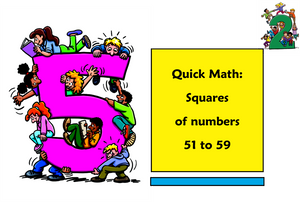## Quick Math: Squaring Numbers 51 to 59

In our previous post, we found a quick shortcut to square two-digit numbers with 5 in the ones place. There is an even simpler shortcut to square two-digit numbers with the digit 5 in the tens place.

For younger students, you can simply teach the trick. For older students explore the Why It Works  section.

The Trick:

To get the thousands and hundreds place value add the digit in the ones place (units) to 25.

To get the tens and ones place, square the number in the ones place. The tens place will be zero for the numbers 51, 52, and 53.

For example, 542 Add the ones digit to 25 to get the thousands and hundreds places. 25 + 4 = 29, which represents 2900.

Square the digit in the ones place to get the digits for the tens and units. 4 x 4 = 16

542 = 2900 + 16 = 2916

Print the Teaching Sheets for the trick. On the first sheet ask students to put in a random digit in the box to complete the two-digit number to square. Follow the steps. Remind students that if the product of squaring the ones digit is a single digit answer, the tens place is zero.

The second page is a practice sheet for the numbers 51 to 59.

Why It Works

Like the previous post, let’s make the tens place X and the units (ones) place Y and solve for variables first. The algebra is the same equation as last time. In fact, this algebraic equation is the same for the square of any two-digit number.

(x + y)2 = (x + y)(x + y) = x2 + xy + xy + y2 = x2 + 2xy + y2

In our example of 542,  x = 50 and y = 4

The 25 that we start with for the thousands and hundreds place is simply x2 .

Since all the numbers from 50 to 59 have the digit 5 in the tens place, it will be 25 for all the numbers. Remember this is actually 50 times 50 which equals 2500.

The step for adding the units digit to 25 comes from the 2xy. We can use the associative property of multiplication to first find the product of 2x and then multiply by y. For all the numbers in the 50's, x=50 Two times that will equal 100. 2(50) = 100.

So the 2xy part of the algebra equation will always yield a product that puts the units digit into the hundreds place.

For our example of 54 x = 50, y = 4, so 2xy = (two times 50) times y. Which is 100 times y.

100 times 4 is 400.

Add that to the  x2 which will be 2500. 2500 + 400 = 2900

Next, add the digits for the tens and units. This is the ypart of the algebra equation. Remember that we designated y as the units place. In our example of 54, the units (ones) place is 4, so we square that number and add it to 2900 for the square are 54.

y= 4 x 4 = 16

54= 2900 + 16 = 2916.

Try it with all the squares that begin with 5 from 50 to 59.

502 = 2500
512 = 2601
522 = 2704
532 = 2809
542 = 2916
552 = 3025
562 = 3136
572 = 3249
582 = 3364
592 = 3481## Thanks for Visting Free Gift:

Practice Multiplication Skills with Multiplication Sliders  Download Here and use the discount code: MathBlog at checkout and receive your PDF file instantly! Offer Expires June 30, 2017

Offer Expires June 30, 2017

Join our e-mail list!Subscribe to this blog's RSS feed using http://feeds.feedburner.com/McruffyPress-McruffyMathBlog## McRuffy Press offers complete math curriculum by grade level, math activity books, manipulatives, games and more! Find more McRuffy Math products here.

Improve multiplication facts skills with our Multiplication Fast Fact books:Multiplication Facts (Book 1) Drills factors from 1 to 10 in our self-checking, non-consumable format that allows students to practice until they have mastered basic facts.Multiplication Practice (Book 2) Drills factors from 1 to 10 in our self-checking, non-consumable format that allows students to practice until they have mastered basic facts.